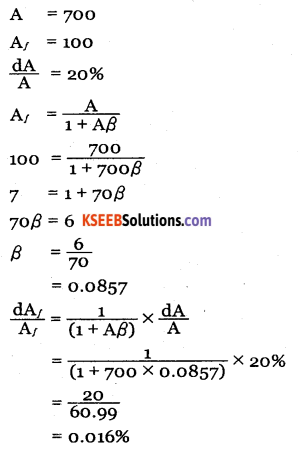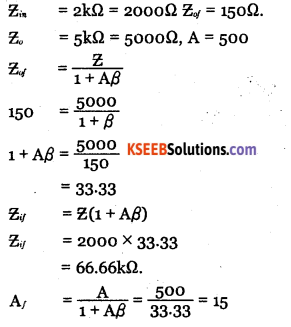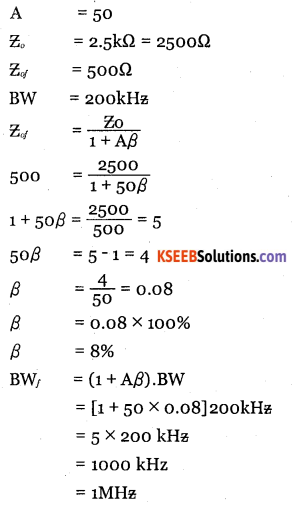# 2nd PUC Electronics Question Bank Chapter 4 Feedback in Amplifiers

Students can Download 2nd PUC Electronics Chapter 4 Feedback in Amplifiers Questions and Answers, Notes Pdf, 2nd PUC Electronics Question Bank with A nswers helps you to revise the complete Karnataka State Board Syllabus and score more marks in your examinations.

## Karnataka 2nd PUC Electronics Question Bank Chapter 4 Feedback in Amplifiers

### 2nd PUC Electronics Feedback in Amplifiers One Mark Questions and Answers

Question 1.
What is feedback?
Feedback is the process of taking a part of output signal and feeding it back to input circuit.

Question 2.
Mention the types of feedback.

• Positive feedback.
• Negative feedback.

Question 3.
Define positive feedback.
Positive feedback is applying the feedback signal in phase with applied input.

Question 4.
Define negative feedback.
Negative feedback is applying the feedback signal 180° out of phase with the applied input.Question 5.
What happens to input impedance of an amplifier when negative feedback is applied? Answer:
Input impedance increases.

Question 6.
What happens to voltage gain of an amplifier when negative feedback is applied?
With negative feedback, gain decreases.

Question 7.
What is meant by loop gain?
The product of open loop gain and feedback ratio is called the loop gain.

Question 8.
What is meant by closed loop gain?
Closed loop gain is the gain of an amplifier with negative feedback.

Question 9.
Define feedback factor?
Feedback factor is the ratio of feedback voltage to output voltage.

Question 10.
Name the type of feedback that is preferred for an amplifier?
Negative feedback.Question 11.
Which type of feedback is required in an oscillator?
Positive feedback.

Question 12.
What is the effect of negative feedback on the bandwidth of an amplifier?
Bandwidth increases.

Question 13.
What is the effect of negative feedback on stability of an amplifier?
Stability increases.

Question 14.
Which type of negative feedback decreases both input and output impedances of an amplifier?
Voltage shunt negative feedback.

Question 15.
Which type of negative feedback increases both input and output impedances of an amplifier?
Current series negative feedback.

Question 16.
Name the type of feedback used in oscillator.
Positive feedback.Question 17.
What is net input to basic amplifier with positive feedback?
Vi = Vs + VF

Question 18.
What is the net input to basic amplifier with negative feedback?
Vi = Vs-VF

Question 19.
How is the gain bandwidth product of an amplifier?
Gain bandwidth of an amplifier is always a constant.

Question 20.
Find output voltage if feedback fraction is 0.2 and feedback voltage is 0.02V?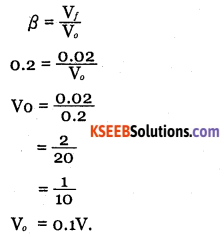Question 21.
Mention four types of negative feedback. Ans. 1. Voltage series negative feedback.

• Voltage shunt negative feedback.
• Current series negative feedback.
• Current shunt negative feedback.### 2nd PUC Electronics Feedback in Amplifiers Two Marks Questions and Answers

Question 1.
Distinguish between open loop and closed loop gain.
Open loop gain of an amplifier is gain of the amplifier without feedback. Closed loop gain of an amplifier is gain of the amplifier with feedback.

Question 2.
What are advantages of negative feedback.

• Stabilisation of the gain.
• Increase in input impedance.
• Decrease in output impedance.
• Increase in bandwidth.
• Reduction in noise and distortion.

Question 3.
Mention the disadvantages of positive feedback.

• Poor gain stability
• More distortion and noise
• Poor frequency response.

Question 4.
Explain the effect of positive and negative feedback on the gain of an amplifier.
With positive feedback, gain of an amplifier increases and with negative feedback, gain an amplifier decreases.

Question 5.
Draw the block diagrams of voltage shunt and current series negative feedback.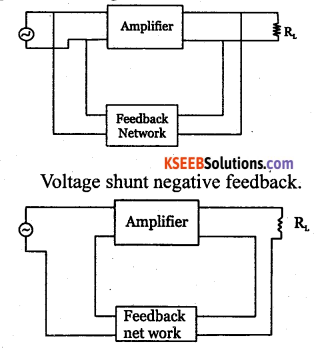Current series negative feedback.

Question 6.
Why is positive feedback seldom used in amplifier?
Positive feedback is seldom used in amplifiers because the positive feedback increases distortion and noise, decreases the gain stability and results in poor frequency response.Question 7.
Name the type of feedback in an amplifier and in an oscillator.

1. Negative feedback is used in an amplifier.
2. Positive feedback is used in an oscillator.

Question 8.
Which type of negative feedback increases the input impedance and decreases output impedance of an amplifier?
Voltage series negative feedback.

Question 9.
An amplifier has a gain of 900. When negative feedback is applied, the gain reduces to 250. Find the feedback fraction.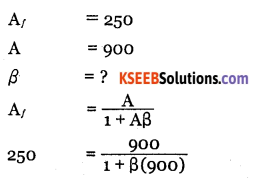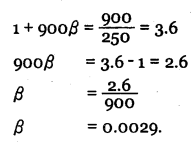Question 10.
An amplifier has a bandwidth of300 KHz and voltage gain of 100. Calculate its bandwidth if 10% negative feedback is introduced.
BW/ = (1 + Aβ)BW
= (1 +100 x 0.1) x 300kHz
= 3,300kHzQuestion 11.
If the bandwidth of an amplifier is 20 kHe and open-loop gain is 200 and β = 0.1, find
the gain of the amplifier with negative feedback.
A= 200,
β = 0.1,
BW = 20 kHz .
Gain with feedback is BWF
= (1+Aβ) BW
= (1+200 x 0.1) x 20 kHz
= 21 x 20 kHz
= 420 kHz                                                                                                  .

Question 12.
Find voltage of an amplifier in dB scale if its voltage gain is 10s.
Gain in dB = 20 log Av
= 20 log 105
= 20 x 5 log10 10
= 100 x 1 = 100 dB.

Question 13.
Find the power gain of an amplifier whose power gain in 50dB.
Gain in dB = 10 log10 A
50 = 10 log10Ap
5 = log10 Ap
log10 Ap = 5
Ap = 105
∴ loga N = x =) ax = N.

Question 14.
Write the expressions for the input voltage
(i) with positive feedback and
(ii) with negative feedback voltage.
(i) V = V + Vf
(ii)Y = Vs – VfQuestion 15.
Mention the four types of negative feedback.

• Voltage series negative feedback.
• Voltage shunt negative feedback.
• Current series negative feedback.
• Current shunt negative feedback.

Question 16.
Write the expressions for
(i) open loop gain
(ii) Closed loop gain
i. Open loop gain, $$A=\frac{V_{o}}{V_{i}}$$
ii. Closed loop gain, $$\mathrm{A}_{f}=\frac{\mathrm{V}_{o}}{\mathrm{V}_{s}}$$

Question 17.
Draw block diagrams to show
(i) current shunt negative feedback and
(ii) voltage series negative feedback.
(i) Current shunt negative feedback.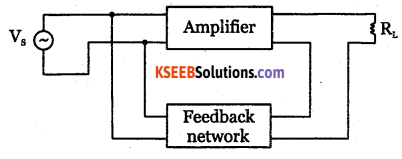(ii) Voltage series negative feedback.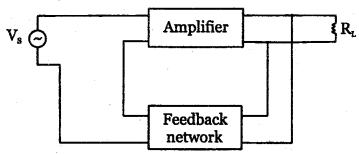Question 18.
Distinguish between positive feedback and negative feedback.

• Positive feedback is the process of applying a portion of output in phase with the input.
• Negative feedback is the process of applying a portion of output out of phase with the input.

Question 19.
Explain how stability is achieved in negative feedback amplifier.
Fluctuation in gain with negative feedback is: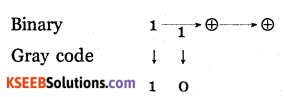For negative feedback, 1+A β> 1 and hence $$A \oplus B=A \bar{B}+\bar{A} B$$ . With negative feedback, fluctuation in the gain decreases and the stability is achieved

### 2nd PUC Electronics Feedback in Amplifiers Three Marks Questions and Answers

Question 1.
Explain the terms feedback factor, loop gain and closed loop gain.

• Feedack factor is the ratio of feedback voltage to the output voltage.
• Loop gain of an amplifier is the product of gain and feedback fraction.
• Closed loop gain is the gain of an amplifier with negative feedback.Question 2.
Draw the frequency response curves of an amplifier with and without negative feedback.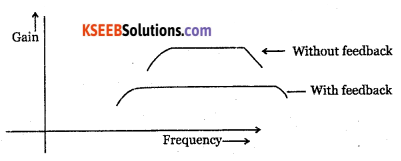Question 3.
Relate the bandwidth of an amplifier with and without feedback and comment on the gain-bandwidth product of an amplifier.
BWf = BW(1 + Aβ)
The gain-bandwidth product of an amplifier is always a constant ie B W x A = BWf x Af

### 2nd PUC Electronics Feedback in Amplifiers Five Marks Questions and Answers

Question 1.
Derive the expression for the gain of an amplifier with negative feedback.
The open loop gain of an amplifier is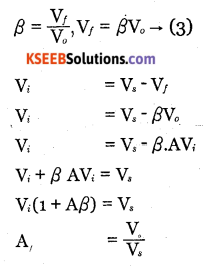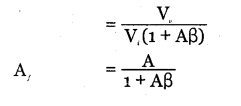Question 2.
Explain the effect of negative feedback on the stability of the gain.
The voltage gain of an amplifier with negative feedback is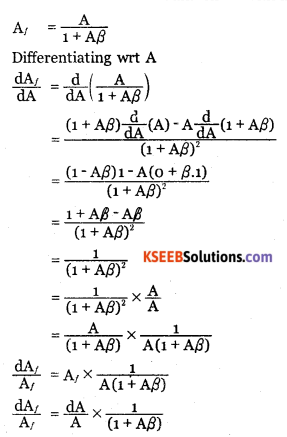As 1+Aβ >1, the percentage change in Af is less than percentage change in A.

Question 3.
Draw the block diagrams for the four types of negative feedback.
1.Voltage series negative feedback.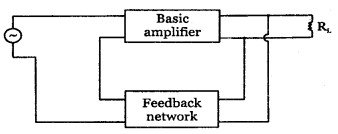2. Voltage shunt negative feedback: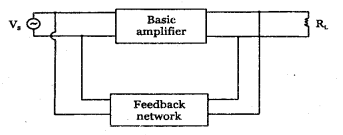3.Current series negative feedback: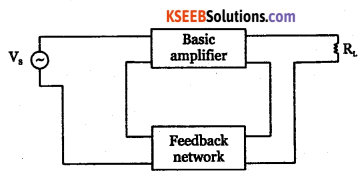4. Current shunt negative feedback: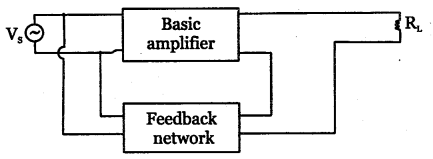Question 4.
Derive an expression for the input impedance of an amplifier with negative feedback.
An amplifier should have high input impedance so that it will not load the preceding stage or input voltage source. High input impedance for an amplifier can be achieved with Voltage series negative feedback.

Let Ii and Vi be input current and input voltage to the basic amplifier. Vs is input to feedback amplifier, Vf is feedback voltage and vo is the output voltage.

Input impedance of basic amplifier is
$$z_{i}=\frac{V_{i}}{I_{i}}-(1)$$

Input impedance of feedback amplifier is
$$z_{v}=\frac{V_{s}}{I_{i}} \rightarrow(2)$$

Input voltage to basic amplifier with negative feedback is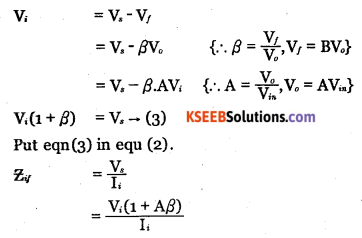$$=\left(\frac{V_{i}}{I_{i}}\right)(1+A \beta)$$
Zif = Zi (1+Aβ)from eq ………… (1)
As (1 + Aβ) > 1 for negative feedback, Zif > Zi
Hence with negative feedback, input impedance of an amplifier increases.

Question 5.
Derive an expression for the output impedance of an amplifier with negative feedback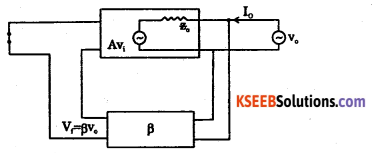An amplifier should have a low output impedance so that it can deliver power to load without much loss. With negative feedback, the output impedance of the amplifier decreases which is desirable.

Let Zo be output impedance of basic amplifier and Zof be the output impedance with negative feedback. A hypothetical source of voltage Vo is applied at the output. By applying KVL to the output loop,

Vo = IoZo +AVi.
With negative feedback, input to basic amplifier with negative feedback is
Vi = Vs – Vf
V= o – Vf
V– Vf
{As input is short circuited, V = o}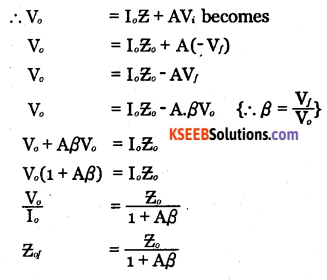As 1+Aβ>1,output impedance of amplifier decreases by a factor(1+Aβ)Question 6.
The input and output voltages of an amplifier are 20 mV and 5V respectively. If the gain with nagative feedback is 100 and input impedance without feedback is 15kΩ, find feedback fraction and input impedance with feedback.
Vin = 20mv = 20 x 10-3V
V0 =5V
Af= 200
Zif = 15kΩ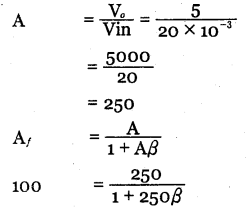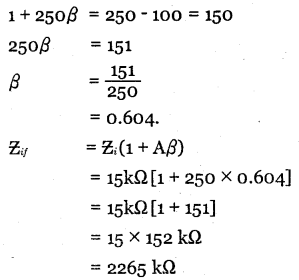Question 7.
An amplifier with loop gain of 25 is, subjected to a negative feedback of 12%. If open loop gain changes by 5% due to temperature rise, find percentage change for gain with feedback. If the gain hand with product is 1,25,000 Hz, Calculate the bandwidth with and without feedback.
Aβ = 25
β = 12%
$$=\frac{12}{100}=0.12$$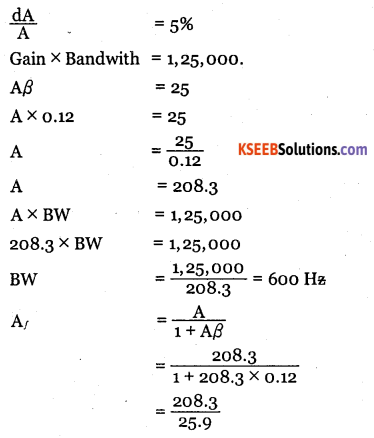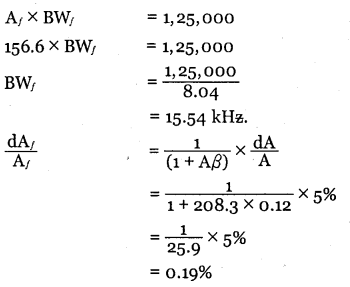Question 8.
The open loop voltage gain of an amplifier is 60 dB. A negative feedback factor 0.03 is applied to it. If the open loop gain change is 10%, find
(a) closed loop gain in decibels
(b) the % change in the over all gain.
G  = 6odB = 20 log A
20 log10 A = 60
log10 A = 3
A = 103 = 1000 {loga N = x = ax = N}Question 9.
The gain of an amplifer falls from 100 to 40 when negative feedback is applied. If the input voltage is IV, calculate (i) feedback factor (ii). Feedback voltage.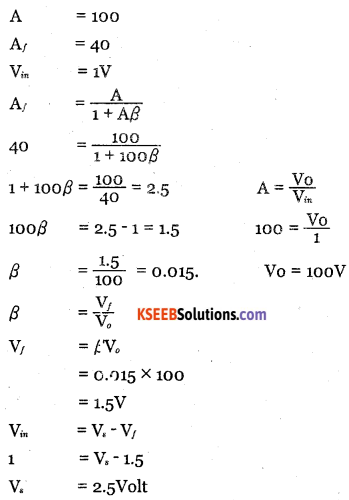Question 10.
An amplifier having a gain of 700 without feedback has overall negative feedback applied which reduces the gain to 100. Calculate feedback factor. If due to ageing of components, the gain without feedback falls by 20%, calculate the percentage fall in gain with feedback.